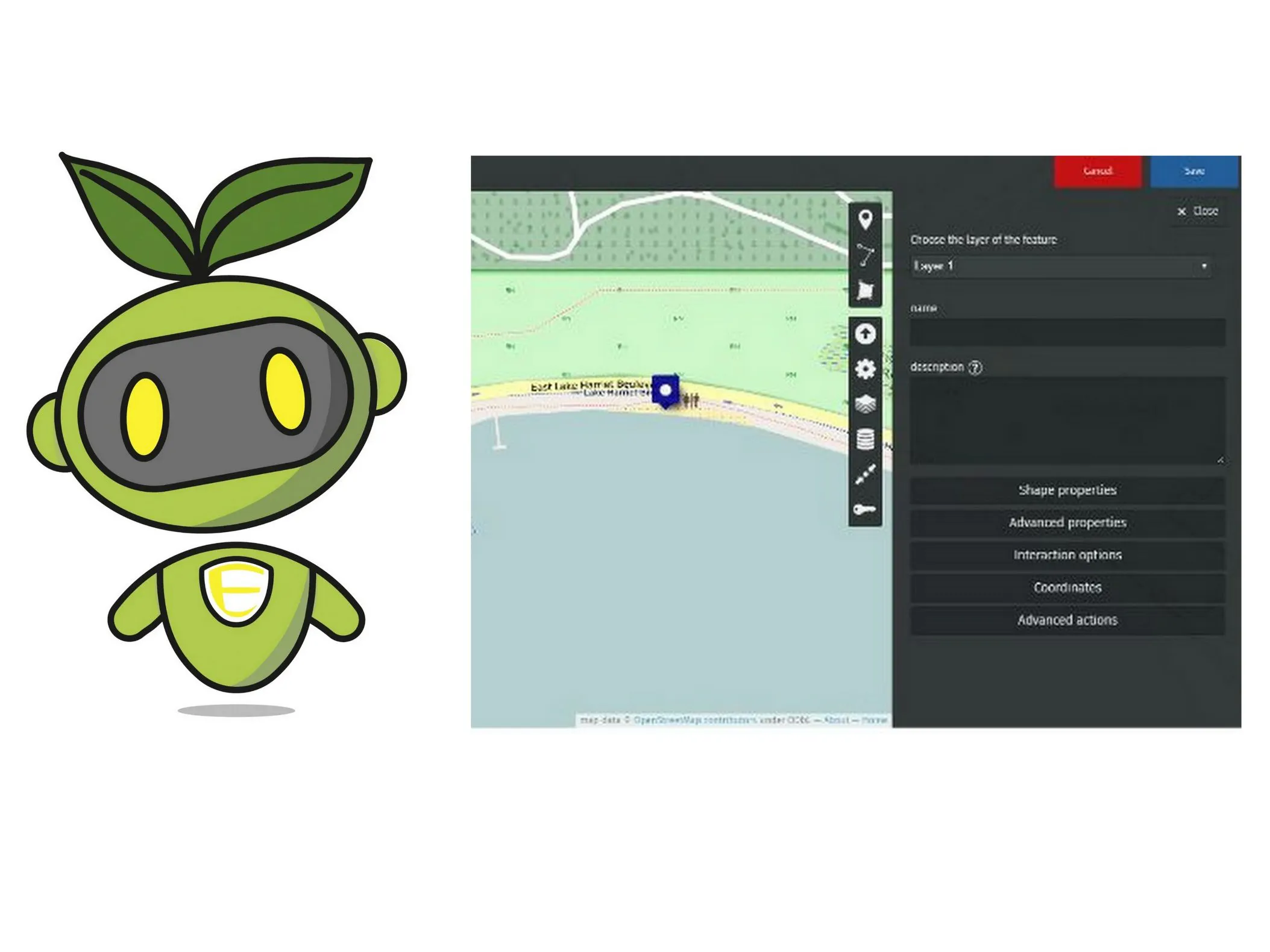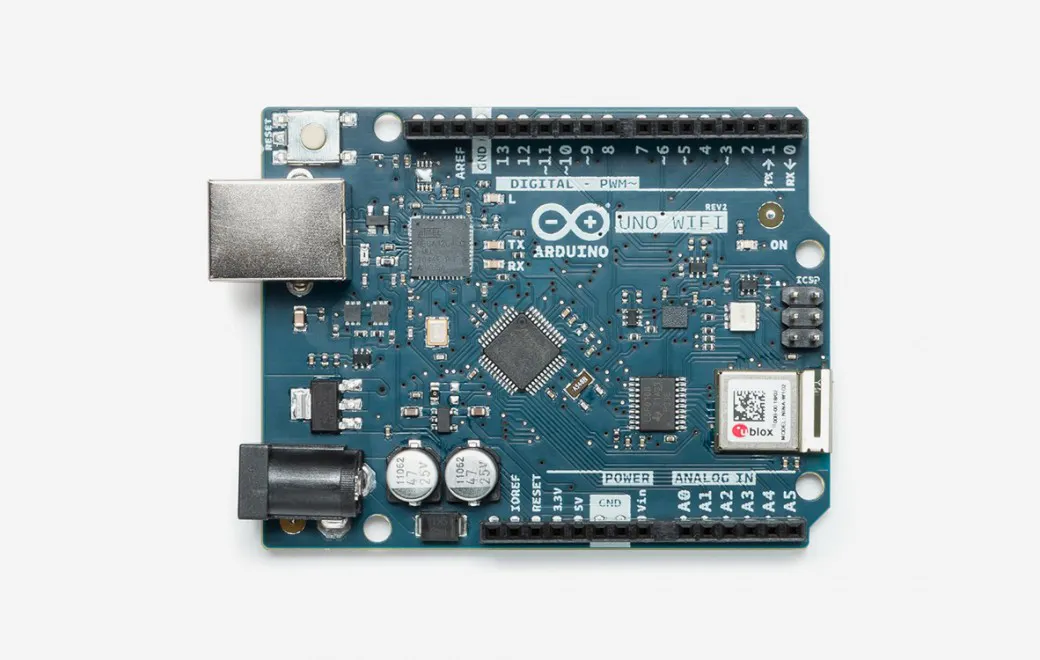# DIY Water Probe and Citizen Science

Learn how to measure water quality, record the data online and launch a citizen science project!

IntermediateFull instructions provided250## Things used in this project

### Hardware componentsArduino UNO Wifi Rev.2
×1
 5x7cm PCB
×1
 Chassis mount binding post Solid core wire
×1Resistor 10k ohm
×1
×1

 uMap

## Code

### Water probe

C/C++
```/*
Water Conductivity Monitor
Sketch for an Arduino gadget that measures the electrical
conductivity of water.
This example code is based on example code that is in the public domain.
*/
const float ArduinoVoltage = 5.00; // CHANGE THIS FOR 3.3v Arduinos
const float ArduinoResolution = ArduinoVoltage / 1024;
const float resistorValue = 10000.0;
int threshold = 3;
int inputPin = A0;
int ouputPin = A5;
void setup()
{
Serial.begin(9600);
pinMode(ouputPin, OUTPUT);
pinMode(inputPin, INPUT);
}
void loop()
{
int analogValue=0;
int oldAnalogValue=1000;
float returnVoltage=0.0;
float resistance=0.0;
double Siemens;
float TDS=0.0;
while(((oldAnalogValue-analogValue)>threshold) || (oldAnalogValue<50))
{
oldAnalogValue = analogValue;
digitalWrite( ouputPin, HIGH );
delay(10); // allow ringing to stop
digitalWrite( ouputPin, LOW );
}
Serial.print("Return voltage = ");
returnVoltage = analogValue *ArduinoResolution;
Serial.print(returnVoltage);

Serial.println(" volts");
Serial.print("That works out to a resistance of ");
resistance = ((5.00 * resistorValue) / returnVoltage) - resistorValue;
Serial.print(resistance);
Serial.println(" Ohms.");
Serial.print("Which works out to a conductivity of ");
Siemens = 1.0/(resistance/1000000);
Serial.print(Siemens);
Serial.println(" microSiemens.");
Serial.print("Total Dissolved Solids are on the order of ");
TDS = 500 * (Siemens/1000);
Serial.print(TDS);
Serial.println(" PPM.");
if (returnVoltage>4.9) Serial.println("Are you sure this isn't metal?");
delay(5000);
}
```

## Credits

### EDUcentrum

0 projects • 4 followers